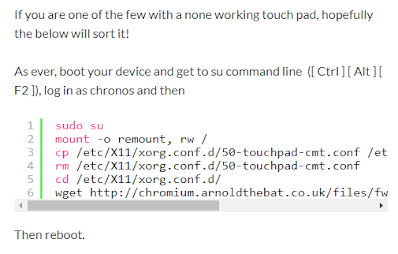## Wednesday, February 29, 2012

### C#: Exploring OpenSSL.NET

I am currently involved in a project module which involves a bit of cryptography. The basic requirements are:
• Generating RSA key pair
• Creating a CSR file
• Generating a Checksum
• Encrypting with private key
• Verifying a signature
So while I was googling for hints, I came across this free library called OpenSSL.NET which looked promising. Find the code below which I use to accomplish the above tasks.

This function generates an RSA key pair and returns a CryptoKey object. The length of the keys are hard-coded to be 2048.
```        /// <summary>
/// Generates a 2048 bit RSA key pair.
/// </summary>
/// <returns>The key container</returns>
public static CryptoKey GenerateRsaKeyPair()
{
using(var rsa = new RSA())
{
rsa.GenerateKeys(2048, 0x10021, null, null);
return new CryptoKey(rsa);
}
}
```

The following function takes in a RSA key and creates a CSR file. I have used a DTO to pass in the additional details needed for the CSR generation.
```        /// <summary>
/// Generates a CSR file content according to the details given.
/// </summary>
/// <param name="csr">CSR details</param>
/// /// <param name="key">RSA key</param>
/// <returns>The CSR file content</returns>
public static string GenerateCsr(CsrProperties csr, CryptoKey key)
{
using (var subject = new X509Name
{
Country = csr.CountryName,
Organization = csr.OrganizationName,
OrganizationUnit = csr.OrganizationalUnitName,
SerialNumber = csr.SerialNumber,
Common = csr.CommonName
})
{
using (var req = new X509Request(0, subject, key))
{
return req.PEM;
}
}
}
```

For Checksum generation, which is also knowing as generating the hash, I used the following code. The algorithm was SHA256.
```        /// <summary>
/// Generates checksum using SHA256 algorithm.
/// </summary>
/// <param name="payLoad">Data to be used for hashing</param>
/// <returns>The hash</returns>
{
using (var algo = new SHA256Managed())
{
}
}
```

To do RSA encryption, I used .NET's native cryptography methods.
```        /// <summary>
/// Encrypts the data using RSA key.
/// </summary>
/// <param name="payLoad">Data to be encrypted</param>
/// <param name="key">RSA key</param>
/// <returns>The encrypted data</returns>
public static byte[] Encrypt(byte[] payLoad, CryptoKey key)
{
using (var rsa = key.GetRSA())
{